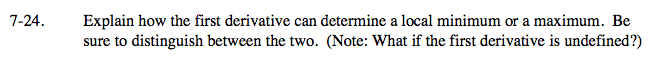### Home > CALC > Chapter 7 > Lesson 7.1.2 > Problem7-24

7-24.

Explain how the first derivative can determine a local minimum or a maximum. Be sure to distinguish between the two. (Note: What if the first derivative is undefined?) Homework Help ✎If the first derivative is f '(x), what is the slope of a maximum or minimum of f(x)?